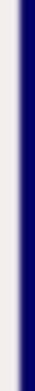homework help forum today's math problem links utilities previous math problem feedback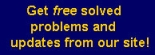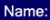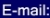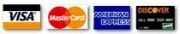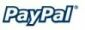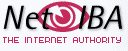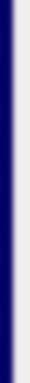# MATH HOMEWORK HELP

6. Decide if f(x) is continuous at x = 3, if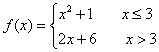Explain the reasoning behind the conclusion

Solution: The function is defined piece wisely, and each piece is continuous. We only have to analyze the point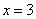. We use side-limits:Therefore, the function is not continuous at. This means that the function is continuous on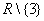.

MGT - Your source of Math and Statistics Homework Help